Get instant live expert help with Excel or Google Sheets“My Excelchat expert helped me in less than 20 minutes, saving me what would have been 5 hours of work!”

#### Post your problem and you’ll get Expert help in seconds.

Your message must be at least 40 characters
Our professional Expert are available now. Your privacy is guaranteed.

# How to Do t Test in Excel

Instead of formulas, we can use Excel Data Analysis Tools to calculate t value in Excel. This functionality is under the tab Data-Data Analysis.

If there is no Data Analysis under the Data tab, we can add it in several steps. Go to File-Options-Add-ins and select Analysis ToolPak. Click button Go, check the Analysis ToolPak check box and click OK.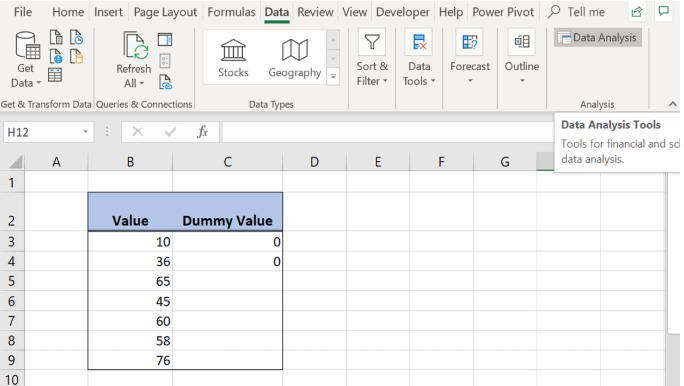Figure 1. Run t Test in Excel

Click on the Data Analysis button, and several t Test options will appear: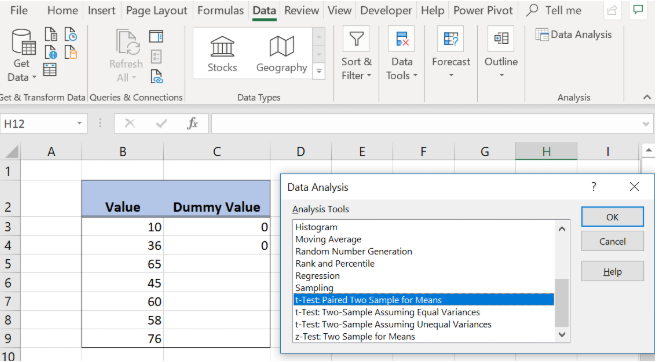Figure 2. How to get tvalue in Excel

## One-Sample t Test in Excel

In the following example, we want to calculate the one sample t test in Excel. We want to check if the column “Value” means is significantly greater than 30. In the Data Analysis tool, there is no built-in option for one sample t test.

Because of that we will create a Dummy column with dummy zero data and choose t-Test: Two-Sample Assuming Unequal Variances: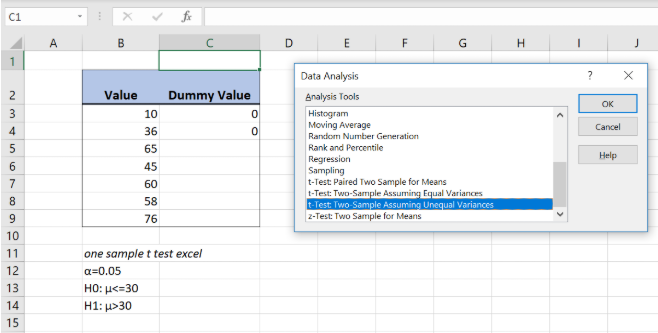Figure 3. One sample t test in Excel

For Variable 1 Range select the data to be tested (in our example column B) and for Variable 2 Range select the Dummy column data. The Hypothesized Mean Difference is number 30 because we want to check if column B means is significantly greater than 30. Select the checkbox Labels and in Output options define the location of the data to be presented.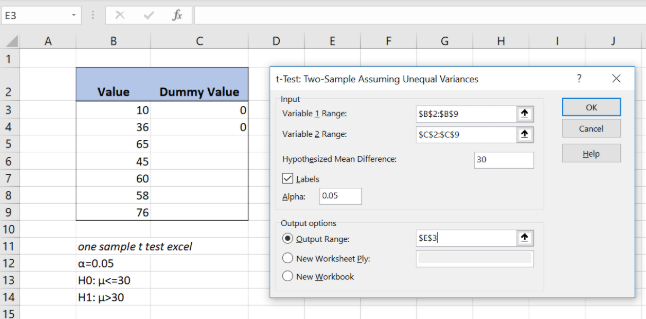Figure 4. One sample t test parameters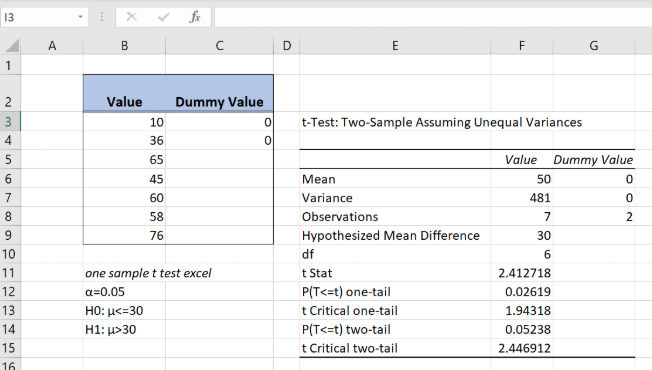Figure 5. The result of the one sample t test in Excel

Since t Stat is greater than t Critical one-tail and P(T<=t) one-tail is less than α, H0 hypothesis is rejected.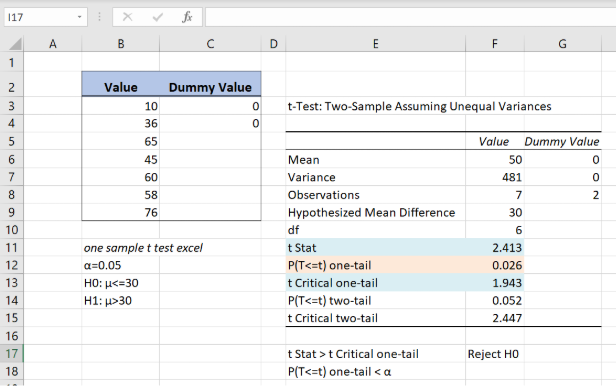Figure 6. Rejected H0 Hypothesis

## Two-Sample t Test in Excel

If we want to calculate two samples paired t test in Excel, in the Data Analysis tab we should choose t-Test: Paired Two Sample for Means.

For Variable 1 Range select the sample 1 range (column B) and for Variable 2 Range select the sample 2 data (column C) while the Hypothesized Mean Difference is 0. Select the checkbox Labels and in Output options define the location of the data to be presented.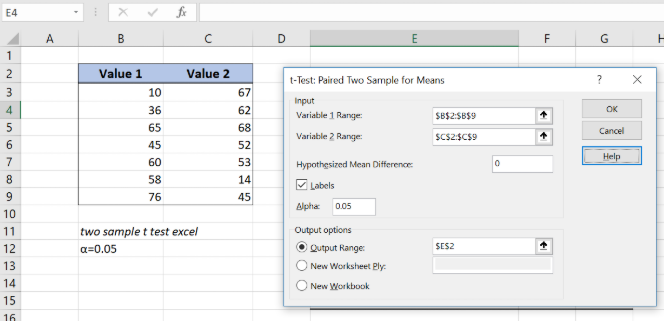Figure 7. Two sample t test excel data inputs

Sample 2 data (column C) while the Hypothesized Mean Difference is 0. Select the checkbox Labels and in Output options define the location of the data to be presented.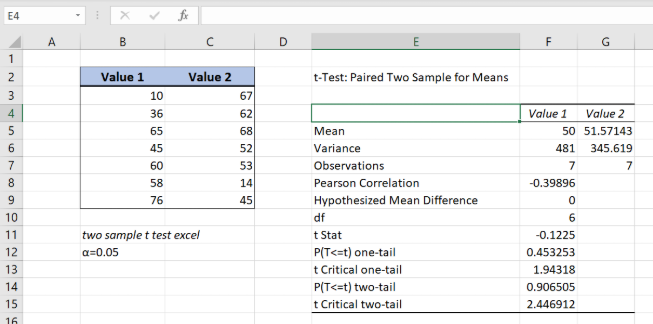Figure 8. Two sample t test excel output

As a result H0 hypothesis is accepted because absolute t Stat value is less than t Critical one-tail.

## How to Do Unpaired t Test in Excel

To do unpaired t test in Excel we should select in the Data Analysis tab t-Test: Two-Sample Assuming Unequal Variances or t-Test: Two-Sample Assuming Equal Variances. In the following example we will do the unpaired t Test in Excel with unequal variances.

For Variable 1 Range we should select column B and for Variable 2 Range column C. The Hypothesized Mean Difference is 0 and alpha is 0.05. After selecting the checkbox Labels we should define in Output options the location of the data to be presented.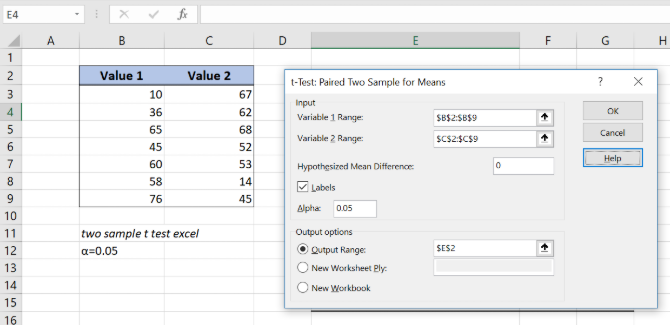Figure 9. Unpaired t test in excel –data inputs

Since absolute t Stat value is less than t Critical one-tail value and H0 hypothesis is accepted: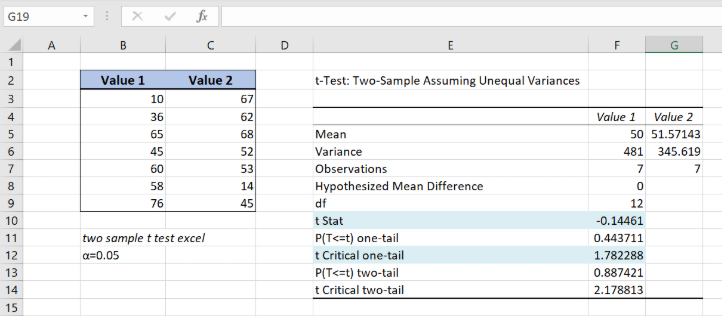Figure 10. Independent t test in excel assuming unequal variances

Most of the time, the problem you will need to solve will be more complex than a simple application of a formula or function. If you want to save hours of research and frustration, try our live Excelchat service! Our Excel Experts are available 24/7 to answer any Excel question you may have. We guarantee a connection within 30 seconds and a customized solution within 20 minutes.